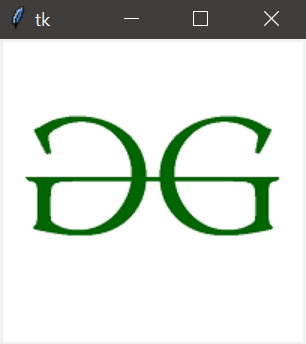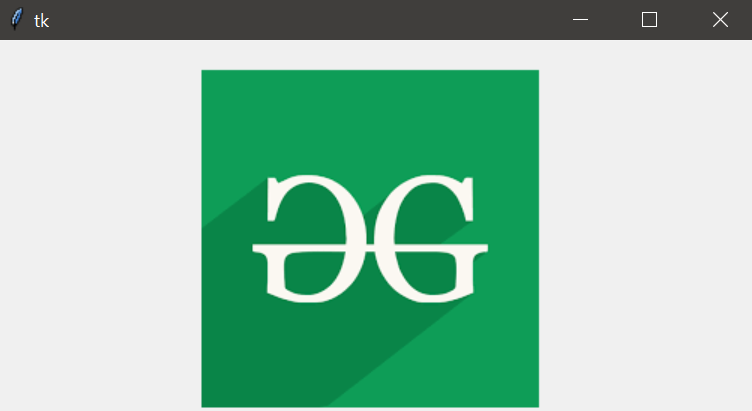Open In App
Related Articles

# Reading Images With Python – Tkinter

There are numerous tools for designing GUI (Graphical User Interface) in Python such as `tkinter`, `wxPython`, `JPython`, etc where Tkinter is the standard Python GUI library, it provides a simple and efficient way to create GUI applications in Python.

In order to do various operations and manipulations on images, we require Python `Pillow` package. If the `Pillow` package is not present in the system then it can be installed using the below command.

• In Command prompt:
`pip install Pillow`
• In Anaconda prompt:
`conda install -c anaconda pillow`

Example 1: The below program demonstrates how to read images with `tkinter` using `PIL`.

 `# importing required packages``import` `tkinter``from` `PIL ``import` `ImageTk, Image``import` `os`` ` `# creating main window``root ``=` `tkinter.Tk()`` ` `# loading the image``img ``=` `ImageTk.PhotoImage(Image.``open``(``"gfg.jpeg"``))`` ` `# reading the image``panel ``=` `tkinter.Label(root, image ``=` `img)`` ` `# setting the application``panel.pack(side ``=` `"bottom"``, fill ``=` `"both"``,``           ``expand ``=` `"yes"``)`` ` `# running the application``root.mainloop()`

Output:In the above program, an image is loaded using the `PhotoImage()` method and then it is read by using the `Label()` method.The `pack()` method arranges the main window and the `mainloop()` function is used to run the application in an infinite loop.

Example 2: Let us look at another example where we arrange the image parameters along with application parameters.

 `# importing required packages``import` `tkinter  ``from` `PIL ``import` `ImageTk, Image  `` ` `# creating main window``root ``=` `tkinter.Tk()  `` ` `# arranging application parameters``canvas ``=` `tkinter.Canvas(root, width ``=` `500``, ``                        ``height ``=` `250``)  `` ` `canvas.pack()  `` ` `# loading the image``img ``=` `ImageTk.PhotoImage(Image.``open``(``"gfg.ppm"``))  `` ` `# arranging image parameters ``# in the application``canvas.create_image(``135``, ``20``, anchor ``=` `NW,``                   ``image ``=` `img) `` ` `# running the application``root.mainloop() `

Output:In the above program, the application parameters are handled by using the `Canvas()` method and the image parameters are handled using `create_image()` method such that the image `gfg.ppm` is displayed in the main window having defined height and width.
Note: The Canvas method create_image(x0,y0, options …) is used to draw an image on a canvas. create_image doesn’t accept an image directly. It uses an object which is created by the PhotoImage() method. The PhotoImage class can only read GIF and PGM/PPM images from files.